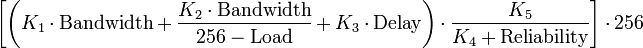## Sunday, December 6, 2009

### CCNP EIGRP Theory

Yesterday I spent most of my time in the CCNP Study Guide book learning about EIGRP in a little more detail. Such things as the metric used for calculating routes:$\bigg [ \bigg ( K_1 \cdot \text{Bandwidth} + \frac{K_2 \cdot \text{Bandwidth}}{256-\text{Load}} + K_3 \cdot \text{Delay} \bigg ) \cdot \frac {K_5}{K_4 + \text{Reliability}} \bigg ] \cdot 256$

EIGRP associates five different metrics with each route:

K1 = Bandwidth modifier

• Minimum Bandwidth (in kilobits per second)

K2 = Load modifier

• Load (number in range 1 to 255; 255 being saturated)

K3 = Delay modifier

• Total Delay (in 10s of microseconds)

K4 = Reliability modifier

• Reliability (number in range 1 to 255; 255 being the most reliable)

K5 = MTU modifier

I also spent time learning about the neighbor table, topology table, and routing tables EIGRP use to determine the best route or Successor route. I spent time going through the 5 types of packets EIGRP uses for making this routing protocol works. Hello's, Updates, Queries, Replies, and ACK (Acknowledgements) are all used in conjunction to make EIGRP work. Today I'm going to be going through many different EIGRP configuration scenarios along with learning more about the DAUL algorithm.